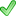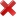# How do I solve exponential equations?Exponential equations in the Earth sciences

## An introduction to solving equations with exponents

Throughout the Earth sciences you will find equations that have exponents where a number is to the power of another number. An exponent shows the number of times the base number is multiplied by itself. So 2^3 is 2 xx 2 xx 2. In this example, "2" is the base and 3 is the exponent. In exponential equations the variable occurs in the exponent. For example, y=ax is exponential equation in which x and y are variables and a is constant. Exponential equations are used to model exponential growth in populations over time and to model exponential decay based on the amount of material present.

## What are examples of exponential equations?

Exponential equations have numerous applications in the sciences. In the Earth sciences two common examples are:

• Exponential growth (used for population or nuclear chain reactions) y=ae^x
• Exponential decay (used to determine the age of an item from radioactive decay or the amount of a reactant in a reservoir) y=ae^(-x)

Solving these types of equations usually requires logarithms.

### How to work with exponents and logarithms

Exponents and logarithms are closely related and can be seen as inverse of each other. Commonly, they are described as "undoing" each other.

• y=a^x is an exponential equation stated as "y equals a to the power of x"
• log_a(y)=x is a logarithmic equation stated as "log base-a of y equals x"
• y=a^x is equivalent to (which means it is the exact same as) log_a(y)=x. Notice that "a" is the base of the exponential and logarithm equations.
The magnetic field example uses base 10, which is also called the common log. Because it is so common, the subscript "10" is rarely written out. Another frequently used log is "e". It is just a number that is equal to 2.71828... and it is called natural log (ln). In some equations and on calculators "e" may be written as "EXP".
As an example, the intensity of the Earth's magnetic field (B) can be described by the equation below:

• B=10^x which is equivalent to
• log_10 B = x

## How do I solve exponential equations?

Below are two examples of some steps to take when you are solving an equation with variables in the exponent.

### Exponential growth

Under the following steps you can find an example in which we determine the time (t) until a population reaches a certain size.

Island County in Washington, which includes the San Juan Islands, has had a 1.3% growth rate since 2000. In 2000, the county had 79,300 residents. In how many years can the county expect to have 100,000 residents?
Use the equation for population growth.

N=N_0 e^(bt)

N is the population; N0 is the starting population; b is the growth rate; and t is time. The letter 'e' is a constant that equals 2.71828....

Step 1. Determine which variables are known and unknown. Usually there is just one unknown.

Step 2. Rearrange the equation to isolate the term with the variable you want to solve for. (For more general help on rearranging equations - check out this page)

Step 3. Because t is in the exponent, use the matching base logarithm to isolate t.

Step 4. Insert the known values into the rearranged equation.

Step 5. Solve for the unknown.

How to use a calculator to solve this equation:

• Most calculators have an "ln" button for natural log. With a graphing calculator you can enter all of the terms on the left-side of the equation, as long as you pay close attention to parentheses.
• Enter: (ln(100000/79300))/0.013 =
• With a phone calculator app, it is possible to follow the text above, but it might be easier to solve the problem in two steps.
• Enter ln(100000/79300) =
• Enter Ans/0.013 =
• In Excel or Sheets, you can use the formula command and again pay attention to parentheses.
• Enter: =ln(100000/79300)/0.013

#### What does the population growth Island County look like on a x-y scatter graph?

On a x-y scatter graph of years vs. population, you can see a graphical example of exponential growth. The line shows the growth rate over time and the dot represents when the population of Island County will reach 100,000 people.

### Thinking more about the math

If the growth rate increased from 1.3% to 2.0%, what would happen to the steepness of the line in the graph above?The higher the growth rate, the steeper the line because the growth rate affects the slope

### Exponential decay

Earth scientists regularly need to determine the age of a rock to place the rock unit or geologic event in an appropriate temporal context. Minerals within rocks preserve ratios of radiogenic isotopes that can be used to calculate when the mineral crystallized or dropped below a certain temperature. Under the following steps you can find an example in which we determine the age of a rock.

Muscovite within a granite originally had 2400 atoms of 40K and no 40Ar. It now has 800 atoms of 40K. Assuming that the only chemical process is radioactive decay of potassium 40K to argon 40Ar, what is the age of the granite? The decay constant, λ, for the K-Ar system is lambda=5.54xx10^(-10)  "1/years"

To determine age, earth scientists use the exponential decay equation, often referred to as the age equation.

N=N_0 e^(-tlambda)

Where N is the amount of isotope present today, N0 is initial amount of isotope, t is time, and λ is the decay constant.

The age equation relies on the decay rate of a radioactive isotope. To describe the rate of decay, scientists use the amount of time required for half of the original material to decay. This time period is called half-life (t_(1/2)).

Step 1. Determine which variables are known and unknown. Usually there is just one unknown.

Step 2. Rearrange the equation to isolate the term with the variable you want to solve for.

Step 3. Because t is in the exponent, use the matching base logarithm to isolate t.

Step 4. Insert the known values into the rearranged equation.

Step 5. Solve for the unknown.

#### What does the exponential decay look like in a table?

On the table of atoms of 40K, time elapsed in millions of years (Ma), and number of half-lives, you can see an example of exponential decay in table form. Notice that the age you calculated in the example problem (1.98 billion years = 1980 million years) is between 1.5-2.0 half-lives for 40K.

## Where do you solve exponential equations in Earth science?

• Environmental science - Population growth
• Geochronology - Dating Earth materials
• Hydrology - Groundwater contamination
• Geophysics - Earthquake moment magnitude and radar wave attenuation
• Atmospheric science - Atmospheric pressure